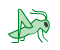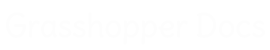﻿ Ivy - Addon for Grasshopper | Grasshopper DocsCommunity documentation for Grasshopper add-ons & plugins# Ivy

##### Ivy is a plug-in for mesh analysis, segmentation and unrolling inside the Grasshopper environment. Ivy uses notions and algorithms from Graph Theory and applies them towards mesh geometry exploration. In order to enable the use of graph theory specific algorithms, a new custom data type was created. The MeshGraph is an abstract construct that combines the geometric characteristics of the mesh with sets of linked data containers. The data containers that can be visualized as the mesh faces are the nodes of the graph and the links between them are the graph edges. The graph edges can be visualized as the mesh topological edges.
 1|Mesh Graph
 2|Weight Graph
 3|Primary Segmentation
 4|Secondary Segmentation
 5|Iterative Segmentation
 6|Special Segmentation
 7|Fabrication
 8|Mesh Info
 9|Other Tools

### 3|Primary Segmentation

 Agents Programmed Behavior (AgentPB)Create a series of trees on the given graph that spread out based on the limits imposed and a programed behavior that switches between two characters Explore and Consume Agents Control Random (AgentsCR)Create a series of trees on the given graph that spread out based on the limits imposed and the behavior given as a chance pick between two characters Explore and Consume DFS Edge Weight (dfsEdge)Compute the a tree from the graph using a Depth first search based on edge weight MST Kruskal concavity (mstCon)Compute the minimum spanning tree for the mesh graph including convex, concave and flat region detection. This uses a modified Kruskal algorithm with weight limits MST Djikstra (mstD)Compute the minimum spanning tree for the mesh graph using Djikstra's algorithm MST Kruskal (mstK)Compute the minimum spanning tree for the mesh graph using a modified Kruskal's algorithm MST Kruskal Valence (mstKv)Compute the minimum spanning tree for the mesh graph using a modified Kruskal's algorithm with max valence preference MST Prim (mstP)Compute the minimum spanning tree for the mesh graph using Prim's algorithm Multi Root MST edge weight (mrMSTedge)Constructs a set of trees based on the given graph Multi Root MST node weight (mrMSTnode)Constructs a set of trees based on the given graph Multi Root MST concavity (mrMSTconc)Constructs a set of trees based on the given graph

### 9|Other Tools

 NodeGeo to Graph (Ng2Gr)Insert the node geometry into a MeshGraph Cull Graph Duplicates (CullGrph)Cull graph duplicates from a graph list. Equality is based on topology Get deepest nodes (DeepestN)Retrieves the deepest nodes in a tree graph. Here the deepest node/nodes are considered the ones that have the longest traceable route to a leaf(edge node) Graph Equality (GraphEqual)Test equality between two mesh graphs. Only topological equality is tested CreaseMesh (CreaseM)Create a set of creases in the faces of the mesh along the edges of the Mesh Graph GraphStructure (GrphStruct)Create a set of lines that approximate the graph and have different offsets from the base mesh according to the number of steps from the root ThinMesh (Thin)Create a thiner mesh based on the connections in the MeshGraph Weave MGraphs (Weave)Weave two MeshGraph segmentations Set Node Geometry (setNGeo)Assign geometry (flat curves) to nodes Set Tree Root (TreeRoot)Set the root of the graph to the specified mesh node

### 2|Weight Graph

 Color Edge Weight (ColorWeight)Assign a weight value to graph edges based on the color averaged for an edge. Values will be normalized to the 0...1 interval Custom Edge Weight (cEdgeWeight)Assign a custom weight to graph edges - Warning ! If you reference mesh edges, naked edges will not be considered. Values are normalized in the 0..1 interval Face Angle Edge Weight (FAWeight)Assign a weight value to graph edges based on the angle between the faces connected by an edge. Values will be normalized to the 0...1 interval Custom Face Weight (cFaceWeight)Assign a custom weight to graph nodes (mesh Faces). Values are normalized in the 0..1 interval Face Size Node(mesh face) Weight (fsFaceWeight)Assign weight to graph nodes based on the area of the mesh faces. Largest faces will have the smallest values. Face Midpoint Distance Edge Weight (MDistWeight)Assign a weight value to graph edges based on the distance between the midpoints of the faces the edge conects. Values will be normalized to the 0...1 interval

### 7|Fabrication

 CustomFlap (cFlp)Create a custom data flap to use for the unroll component Flat Fabrication (FlatFab)Takes care of the fabrication preparation for the parts Flat Fabrication Multi (FlatFabM)Takes care of the fabrication preparation for the parts. Special version for dealing with complex data structures. Use especially in conjunction with multiple weaved strands SimpleFlap (sFlp)Create a standard simple data flap to use for the unroll component

### 1|Mesh Graph

 Graph Edges (Edges)Outputs the relevant information (geometric and numeric) stored in the MeshGraph object edges Graph Nodes (Nodes)Outputs the relevant information (geometric and numeric) stored in the MeshGraph object nodes Graph from Mesh (GrphMsh)Creates the graph correspondence of a mesh. Faces will be nodes in graph and the mesh topological edges will be the edges in the graph Graph to Mesh (Grph2Msh)Creates the mesh from the graph nodes(faces) and edges. Only the nodes still present in the graph will be referenced back into mesh faces.

### 8|Mesh Info

 EdgeAngle (eAngle)Extracts the dihedral angle of an edge. Only the edges in the graph are used EdgeTraversal (eTrav)Calculates the traversal distance for an edge of the graph. Here it is the lenght of the polyline connecting the face centers and edge midpoint EdgeTypes (mEt)Extracts the edges of the base mesh for the graph based on their use in the graph. Graph Edge or Cut Edge Orange PEEL Edges (OPE)Gets a set of edges in the MeshGraph that separate peel layers

### 6|Special Segmentation

 MeshGraph Unroll (mgUroll)Unroll the underlying mesh of a tree graph. MeshGraph Visual Unroll (mgUrollViz)Unroll the underlying mesh of a tree graph on the object itself as a from finding technique. Shortest Paths in a weighted mesh graph (SPath)Compute the shortest (Cheapest) path between 2 nodes in a mesh graph using Djikstra's algorithm. Calculation uses edge weight.

### 4|Secondary Segmentation

 Weight Deviation Split Graph (DevSplit)Splits a mesh graph in subgraphs based on whether edge weight is larger than next edge in tree hierarchy Weight Split Graph (WSplit)Splits a mesh graph in subgraphs based on whether edge weight falls in the supplied interval

### 5|Iterative Segmentation

 K-Means Clustering (kMeans)Splits a mesh graph (not a tree) in an iterative fashion based on a number of parts specified by the user

## Video Tutorials

Site design © Robin Rodricks.   Ivy and associated data © 2023 Andrei Nejur.
Rhinoceros and Grasshopper are registered trademarks of Robert McNeel & Associates.  Hosted by GitHub